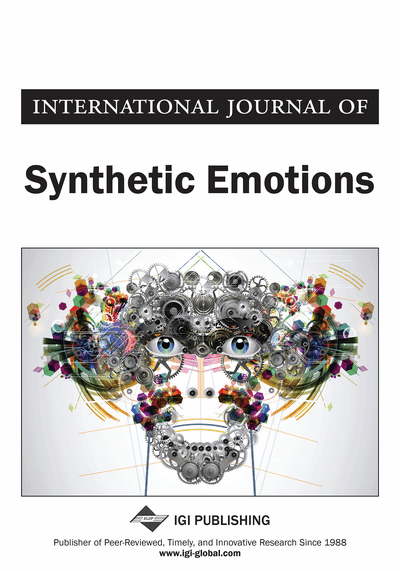# On Integration Linguistic Factors to Fuzzy Similarity Measures and Intuitionistic Fuzzy Similarity Measures

Pham Hong Phong, Vu Thi Hue
DOI: 10.4018/IJSE.2019010101
OnDemand:
(Individual Articles)
Available
\$37.50
No Current Special Offers

## Abstract

The article is concerned with integrating linguistic elements into fuzzy similarity measures and intuitionistic fuzzy similarity measure. Some new concepts are proposed: a fuzzy linguistic value (FLv), a fuzzy linguistic vector (FLV), an intuitionistic fuzzy linguistic vector (ILV) and similarity measures. The proposed measures are used to build classification algorithms. As predicted theoretically, experiments show that with the same type of similarity measures, the linguistic-aggregated similarity measures produce better results in classification problems.
Article Preview
Top

## 1. Introduction

This section covers general knowledge of fuzzy similarity measure, intuitionistic fuzzy similarity measure, linguistic similarity measure and linguistic vector similarity measure. Some of the most basic similarity measures are mentioned. In order to make it possible to compare new measurements with old measurements, based on the expression, we classify similarity measures as follows:

• Type 1: Hamming-distance-based similarity measures

• Type 2: Min-max-based similarity measures

• Type 3: Exponent-hamming-based similarity measures

• Type 4: Exponent-square-root-based similarity measures

### 1.1. Fuzzy Similarity Measures and Intuitionistic Fuzzy Similarity Measures

The similarity measure is an important mathematical concept that is used to estimate the degree of similarity between objects and to be applicable in pattern recognition problems such as clustering, classification and information retrieval. In the case of inadequately defined and ambiguous objects, instead of the traditional similarity measure, fuzzy similarity or intuitionistic fuzzy similarity measures should be used.

#### 1.1.3. Definition 3

It is convention that if the denominator is 0, the fraction is equal to 1.

#### 1.1.4. Definition 4

It is convention that if the denominator is 0, the fraction is equal to 1.

Some typical application of fuzzy similarities are: image processing (Bloch 1999; Weken et al., 2005; Weken et al., 2004), fuzzy reasoning (Wang et al., 2008), shape retrieval using the SQUID data set described with Fourier descriptor (Gadi et al., 1999), shape classification (Baccour et al., 2007), handwritten Arabic sentences recognition (Baccour & Alimi 2010; Baccour & Alimi 2013) and medical diagnosis (Son & Phong, 2016).

## Complete Article List

Search this Journal:
Reset
Volume 11: 2 Issues (2020)
Volume 10: 2 Issues (2019)
Volume 9: 2 Issues (2018)
Volume 8: 2 Issues (2017)
Volume 7: 2 Issues (2016)
Volume 6: 2 Issues (2015)
Volume 5: 2 Issues (2014)
Volume 4: 2 Issues (2013)
Volume 3: 2 Issues (2012)
Volume 2: 2 Issues (2011)
Volume 1: 2 Issues (2010)
View Complete Journal Contents Listing